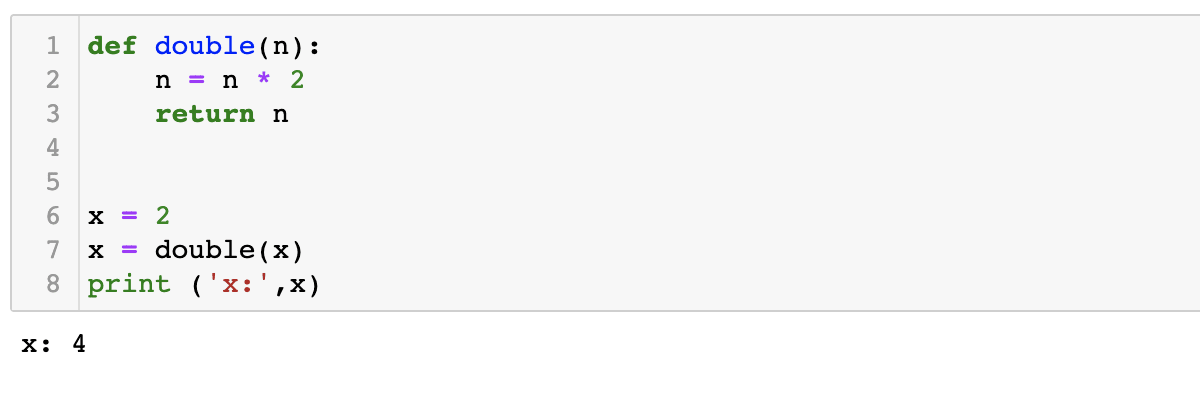Skip to content

# Functions

Functions are a set of related statements that perform a task. They are defined outside of the main block of code. When required, functions can be used or ‘called’ in a code. Defining a function to perform a task helps avoid redundancy and improves the readability of the script.

## Function Definition Syntax

Functions are defined following several conventions. The following is specific to python.

Firstly, the function must be defined. The name of the function is specified after `def`. To use the function, you may call the name of the function and supply an argument that the function acts on.

Example 1: The function ‘double’ is defined. It takes a number, multiplies the number by 2 and returns the output. The function is applied on the variable ‘x’.

```def double(n):
n = n * 2
return n

x = 2
x = double(x)
print ('x:',x)```Example 2: In this example, the function accepts two arguments, ‘s’ and ‘exclaim’. The first input is a string and the second is a boolean. Whatever the first input is will be printed three time. The second input can have the values of 0 or 1. If the second input is 1, then the 3 exclamation marks will be printed. If the second input is 0, then no exclamation marks will be printed.

```def repeat(s, exclaim):
"""
Returns the string 's' repeated 3 times.
If exclaim is true, add exclamation marks.
"""
result = s + s + s # can also use "s * 3" which is faster (Why?)
if exclaim:
result = result + '!!!'
return result

print(repeat('s',1))
print(repeat('s',0))```Notice also how the lines that make up the function or if-statement in both examples are grouped by all having the same level of indentation.

The `def` keyword defines the function with its parameters. The function name is followed by a set of parentheses, which define how the input should look like. The code within the function is indented. The first line of a function can be a documentation string (“docstring”) that describes what the function does. The docstring can be a single line, or a multi-line description as in the example above. (Yes, those are “triple quotes,” a feature unique to Python!) Variables defined in the function are local to that function, so the “result” in the above function is separate from a “result” variable in another function. The `return` statement can take an argument, in which case that is the value returned to the caller.